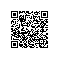# 在SQLServer中区分大小写的几种方法

ALTER TABLE tb
ALTER COLUMN colname nvarchar(100) COLLATE Chinese_PRC_CI_AS
--不区分大小写
ALTER TABLE tb
ALTER COLUMN colname nvarchar(100) COLLATE Chinese_PRC_CS_AS
--区分大小写
alter database 数据库 COLLATE Chinese_PRC_CS_AS

--创建如下用户自定义函数(UDF)

CREATE FUNCTION StrComp(@Str1 VARCHAR(50),@Str2 VARCHAR(50))
--ALTER FUNCTION StrComp(@Str1 VARCHAR(50),@Str2 VARCHAR(50))
RETURNS INTEGER
AS
BEGIN
DECLARE @i INTEGER
--DECLARE @Str1 VARCHAR(50)
--DECLARE @Str2 VARCHAR(50)
DECLARE @y INT
--SET @Str1='a'
--SET @Str2='A'
SET  @i=0
--SELECT ASCII(SUBSTRING(@Str1,@i+1,1))
SET @y=1
DECLARE @iLen INT
SET @iLen = LEN(LTRIM(RTRIM(@Str1)))
IF LEN(LTRIM(RTRIM(@Str1))) < LEN(LTRIM(RTRIM(@Str2))) --THEN
SET @iLen = LEN(LTRIM(RTRIM(@Str2)))
WHILE (@i < @iLen)
BEGIN
IF (ASCII(SUBSTRING(@Str1,@i+1,1))=ASCII(SUBSTRING(@Str2,@i+1,1))) --THEN
SET @i = @i +1
ELSE
BEGIN
SET @y=0
BREAK
END
END
RETURN @y
END

select *
from Table1
Where dbo.StrComp(Field1,'aAbB') =1

SQL Server 数据库中的文本信息可以用大写字母、小写字母或二者的组合进行存储。例如，姓氏可以"SMITH"、"Smith"或"smith"等形式出现。

select * from servers where convert(varbinary, name)=convert(varbinary, N'RoCKEY')

ascii('a')再配合Substring()一起用使用钉钉扫一扫加入圈子
+ 订阅